# Riemann method

Riemann–Volterra method

A method for solving the Goursat problem and the Cauchy problem for linear hyperbolic partial differential equations of the second order in two independent variables (cf. Hyperbolic partial differential equation),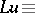(1)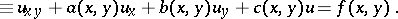In Riemann's method a fundamental role is played by the Riemann functionwhich, under suitable conditions on the coefficients,,, and, is defined as the solution of the particular Goursat problem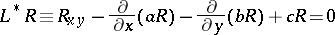with the characteristic boundary conditions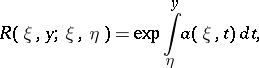With respect to the variables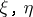, the functionis a solution of the homogeneous equation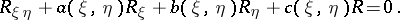When,, one has, where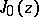is the Bessel function of order zero.

The Riemann function may also be defined as the solution of the weighted integral Volterra equation:(2)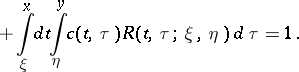The Riemann method for solving the Goursat problem is as follows: For any functionthat can be differentiated to the corresponding order, the following identity is valid: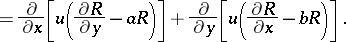Integrating over the rectangle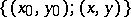and integrating by parts yields that any solutionof (1) is a solution of the weighted integral equation:(3)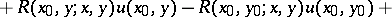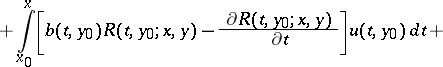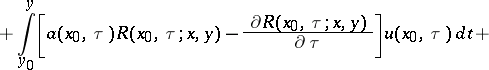Equation (3) demonstrates directly the well-posedness of the Goursat problem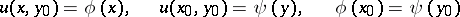for equation (1).

Riemann's method solves the Cauchy problem for equation (1) with initial data on any smooth non-characteristic curve by finding a Riemann function. It thus affords the possibility of writing the solution of this problem in the form of quadratures.

Riemann's method has been generalized to a broad class of linear hyperbolic partial differential equations and systems.

In the case of a linear hyperbolic system of partial differential equations of the second order,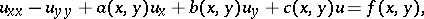where,andare given square, real, symmetric matrices of order,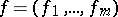is a given, and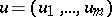is the unknown vector, the Riemann matrix is unambiguously defined as the solution of a system of weighted Volterra integral equations of the form (2) whose right-hand side is the identity matrixof order.

V. Volterra was the first to generalize Riemann's method to the wave equation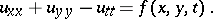(4)

The functionwhere, acts as the Riemann function, which permits that the solution of the Cauchy problem with initial data on the plane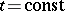and of the Goursat problem with data on a characteristic cone for equation (4) may be written in the form of quadratures.

The method was proposed by B. Riemann (1860).